Coefficient of Variation - Measures of Dispersion, Business Mathematics & Statistics

# Coefficient of Variation - Measures of Dispersion, Business Mathematics & Statistics - Business Mathematics and Statistics - B Com

VARIANCE :

The square of the Standard Deviation is known as Variance.

COEFFICIENT OF VARIATION : It is the ratio of the Standard Deviation to the Mean expressed as percentage. This relative measure was first suggested by Professor Kari Pearson. According to him, coefficient is the percentage variation in the Mean, while Standard Deviation is the total variation in the Mean.

Symbolically

Coefficient of variation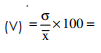Coefficient of stand. deviation × 100.

Note : The coefficient of variation is also known as coefficient at variability. It is expressed as percentage.

Example 55 : If Mean and Standard deviation of a series are respectively 40 and 10, then the coefficient of variations would be 10 / 40 × 100 = 25%, which means the standard deviation is 25% of the mean.

Example 56: An analysis of the monthly wages paid to workers in two firms, A and B, belonging to the same industry gives the following results :

Firm A              Firm B
No. of wage-earners                                           586                   648
Average monthly wages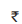52.547.5
Variance of distribution of wages                        100                    121

(a) Which firm A and B pays out the largest amount as monthly wages? (
b) Which firm A and B has greater variability in individual wages?
(c) Find the average monthly wages and the standard deviation of the wages of all the workers in two firms A and B together.

Solution :

(a) For firm A : total wages = 586 × 52.5 =30,765. For firm B : Total wages = 648 × 47.5 =30,780. i.e. Firm B pays largest amount.
(b) For firm A : σ2 = 100 ∴ σ = 10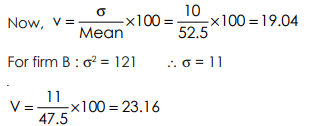∴ Firm B has greater variability, as its coefficient of variation is greater than that of Firm A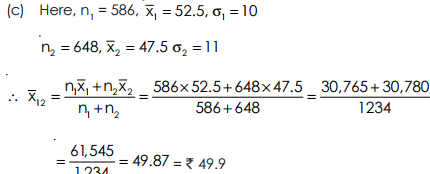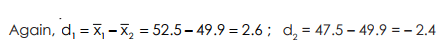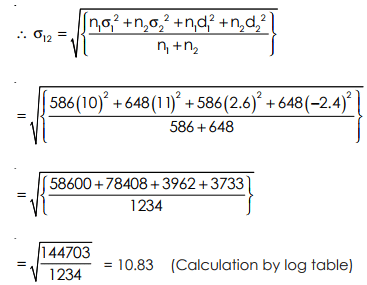Example 57 : In an examination a candidate scores the following percentage of marks :
English     2nd language       mathematics     Science         Economics
62                  74                       58                       61                     44

Find the candidates weighted mean percentage weighted of 3, 4, 4, 5 and 2 respectively are allotted of the subject. Find also the coefficient of variation.

Solution: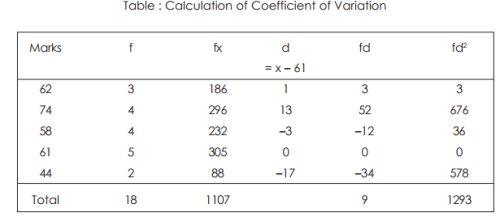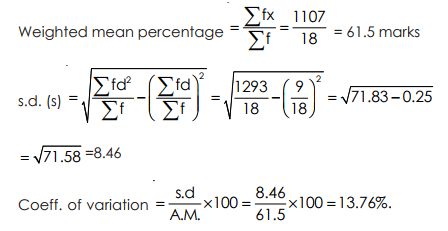Example 58 : The A.M. of the following frequency distribution is 1.46. Find f1 and f2 .
No. of accidents :         0      1      2    3    4      5      total
No. of days :                46       f1  f2    25  10    5      200

Also find coefficient of variation.

Solution:

Putting these values of f1 and f2 we find the following distribution: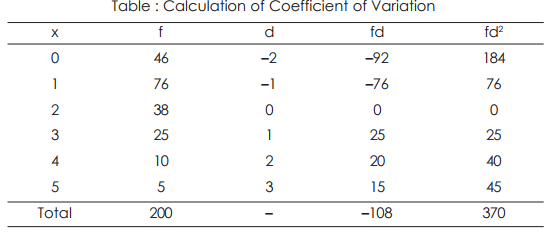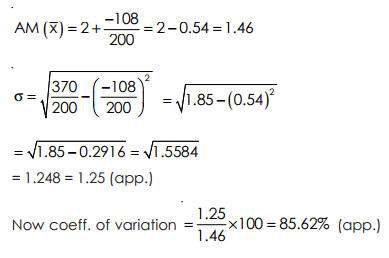1. Standard deviation is based on all the observations and is rigidly defined.
2. It is amenable to algebraic treatment and possesses many mathematical properties.
3. It is less affected by fluctuations of sampling than most other measures of dispersion.
4. For comparing variability of two or more series, coefficient of variation is considered as most appropriate and this is based on standard deviation and mean.

1. It is not easy to understand and calculate.
2. It gives more weight to the extremes and less to the items nearer to the mean, since the squares of the deviations of bigger sizes would be proportionately greater than that which are comparatively small. The deviations 2 and 6 are in the ratio of 1 : 3 but their squares 4 and 36 would be in the ratio of 1 : 9.

Uses of Standard Deviation : It is best measure of dispersion, and should be used wherever possible.

The document Coefficient of Variation - Measures of Dispersion, Business Mathematics & Statistics | Business Mathematics and Statistics - B Com is a part of the B Com Course Business Mathematics and Statistics.
All you need of B Com at this link: B Com

115 videos|142 docs

## FAQs on Coefficient of Variation - Measures of Dispersion, Business Mathematics & Statistics - Business Mathematics and Statistics - B Com

 1. What is the coefficient of variation and how is it calculated?The coefficient of variation is a statistical measure that is used to measure the relative variability or dispersion of a dataset. It is calculated by dividing the standard deviation of the dataset by the mean of the dataset and then multiplying the result by 100 to express it as a percentage.
 2. How is the coefficient of variation useful in business and statistics?The coefficient of variation is useful in business and statistics as it provides a standardized measure of variability that allows for the comparison of different datasets with different units of measurement. It is particularly useful when comparing datasets with different means, as it allows for a better understanding of the relative variability between them.
 3. What does a high coefficient of variation indicate?A high coefficient of variation indicates a high degree of variability or dispersion in the dataset. This means that the individual data points in the dataset are spread out over a wide range of values in relation to the mean. It suggests that there is a greater degree of volatility or uncertainty associated with the dataset.
 4. Can the coefficient of variation be negative?No, the coefficient of variation cannot be negative. Since it is calculated by dividing the standard deviation by the mean and multiplying by 100, it will always be a positive value. If the standard deviation is zero or the mean is zero, the coefficient of variation will be undefined rather than negative.
 5. How can the coefficient of variation be used to compare the variability of different datasets?The coefficient of variation can be used to compare the variability of different datasets by calculating it for each dataset and then comparing the values. A lower coefficient of variation indicates a lower degree of variability, while a higher coefficient of variation indicates a higher degree of variability. By comparing the coefficients of variation, one can determine which dataset has a greater or lesser degree of dispersion.

115 videos|142 docsExplore Courses for B Com examSignup to see your scores go up within 7 days! Learn & Practice with 1000+ FREE Notes, Videos & Tests.
10M+ students study on EduRev
Track your progress, build streaks, highlight & save important lessons and more!
Related Searches

,

,

,

,

,

,

,

,

,

,

,

,

,

,

,

,

,

,

,

,

,

,

,

,

;